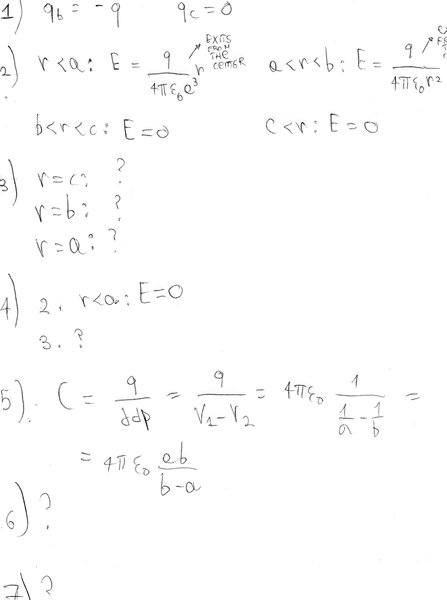# Charged sphere and charged conducting shell

• pedro97

## Homework Statement

A + q = 5 pC charge is uniformly distributed on a non-conducting sphere of radius a= 5 cm , which is placed in the center of a spherical conducting shell of inner radius b = 10 cm and outer radius c = 12 cm. The outer conducting shell is charged with a -q charge. Determine:
1) the charges on the inner and outer surfaces of the shell;
2) the electric field (module, direction) everywhere;
3) the electrostatic potential on the external surface of the conducting shell (r = c), on the internal surface of the shell (r = b) and on the outer surface of the internal sphere of radius a (r = a).
Suppose now to replace the inner sphere with a spherical conductor of radius a charged with the same + q charge:
4) Which of the previous answers will change and how?
5) Determine the potential everywhere;
6) Determine the capacitance of the spherical capacitor formed from the internal conducting sphere of radius a and the outer conducting shell;
7) If a proton (m = 1.67·10-27 Kg) starts from rest from the spherical conductor of radius a, which will be its speed when it hit the inner surface of the outer spherical shell?

/

## The Attempt at a SolutionSo, I would like to know if my attempt to solve the problem is correct, and how can I continue it in the parts I didn't manage to solve...
Thank you!

I would like to know if my attempt to solve the problem is correct,
No.

For the first point my reasoning is: since on the inner sphere we have a + q charge, because of the induction phenomenon, the inner surface of the outer shell has to "balance" that charge, and thus has a charge -q. Since the problem statement tells us that -q is the total shell charge, we know that there is no charge on its outer surface...

Seems to me his answer to (1) is correct.
Placing gaussian sphere at b < r < c forces qb = -q.
Placing gaussian sphere at r > c forces qc = 0.

•SammyS
3) the electrostatic potential on the external surface of the conducting shell (r = c), on the internal surface of the shell (r = b) and on the outer surface of the internal sphere of radius a (r = a).
You can just integrate the E field using your data from (2).

For the first point my reasoning is: since on the inner sphere we have a + q charge, because of the induction phenomenon, the inner surface of the outer shell has to "balance" that charge, and thus has a charge -q. Since the problem statement tells us that -q is the total shell charge, we know that there is no charge on its outer surface...
My mistake - I read it as +5q instead of +q = 5pC.

I don't understand your attempt at 5). Looks more like an attempt at 6).

Last edited:
You can just integrate the E field using your data from (2).
Yes, but we're not told where to set the zero potential. The usual convention is to set that at infinity.

Yes, but we're not told where to set the zero potential. The usual convention is to set that at infinity.
Yes, and that is where he should and can integrate the E field from. What's the problem?

The custom is to set potential to zero at infinity unless that is impossible - as with a charged wire of infinite length.

Yes, and that is where he should and can integrate the E field from. What's the problem?